##### PostgreSQL Aggregate Functions With Practical Examples

By using PostgreSQL aggregate functions, we can compute/calculate a set of rows. These functions perform calculations on the table rows and return only a single row. The Postgres aggregate functions are listed below:

- SUM() Function.

- COUNT() Function.

- AVG() Function.

- MAX() Function.

- MIN() Function.

This write-up will discuss each of the functions mentioned above through Practical examples. So, let's begin.

How to Use SUM() Function in Postgres?

PostgreSQL provides a built-in SUM() function that is used to perform the addition on a set of values. The below snippet illustrates the syntax of the SUM() function:

`SUM(exp);`

Here, “exp” represents an expression.

Example: How to Use SUM() Function on Table’s Data?

Let’s say we have a table bike_details. We will execute the SELECT query to fetch all the records of the bike_details table:

`SELECT * FROM bike_details;`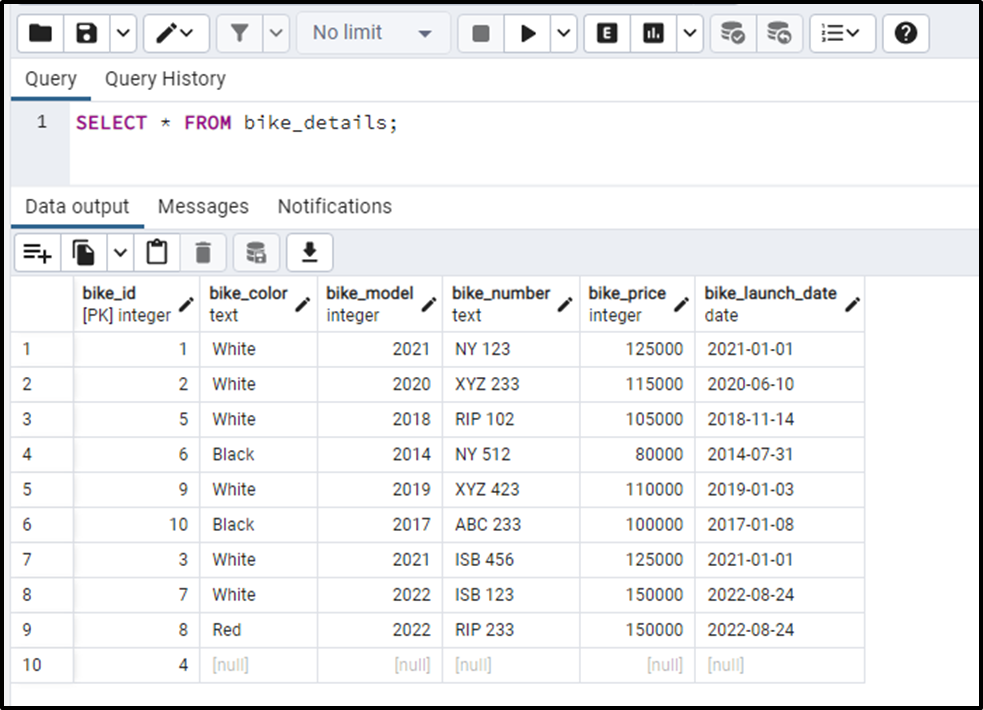Let’s perform addition on the bike_price column using the SUM() Function:

```SELECT SUM(bike_price) AS total_price
FROM bike_details;```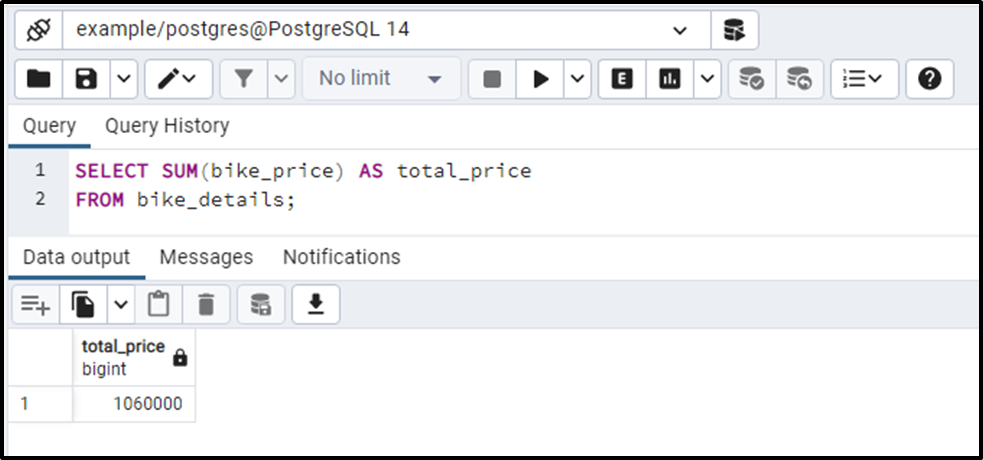The output authenticates that the SUM() function returns the sum of the bike_price column.

How to Use COUNT() Function in PostgreSQL?

The table rows can be counted using PostgreSQL's COUNT() function. In PostgreSQL, the COUNT() function is used to get all the rows, including duplicates and NULL. The below snippet illustrates the syntax of the Postgres COUNT(*) function:

```SELECT COUNT(*)
FROM tab_name;```

COUNT(*) function will fetch all the rows(including duplicates and NULL) from the targeted table based on the specified condition.

Example: How Does the COUNT(*) Function Work in PostgreSQL?

The bike_details table has some duplicated and null values. The following query will calculate the total number of rows in the bike_details table:

```SELECT COUNT(*)
FROM bike_details;```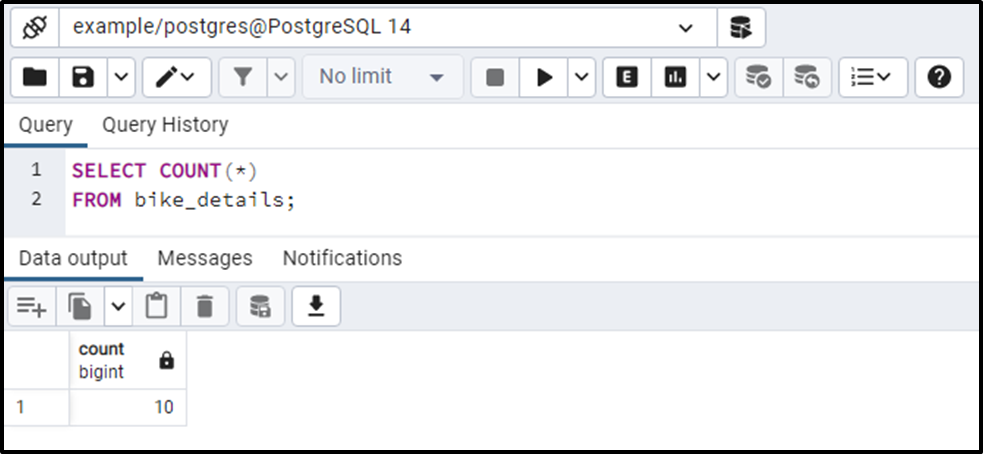The output shows that the bike_details table has 10 rows. It proves that the COUNT(*) function counted all the rows, including the duplicates and null.

How to Use AVG() Function in Postgres?

PostgreSQL provides a built-in AVG() function that is used to retrieve the average of a set. The below snippet demonstrates the basic syntax of the AVG() function:

`AVG(col_name);`

Let’s implement the AVG() function practically to get profound knowledge.

Example: How to Use AVG() Function on Table’s Data?

Let’s compute the average of the bike_price column using the AVG() Function:

```SELECT AVG(bike_price)
FROM bike_details;```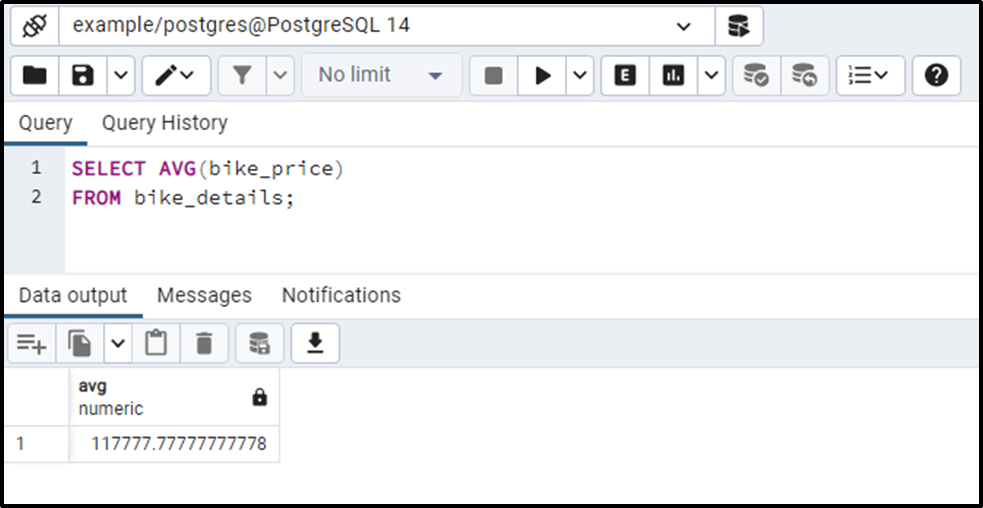Let’s run the below query to get the average up to specific decimal places:

```SELECT AVG(bike_price)::numeric(10, 3)
FROM bike_details;```

The above query will return the average of bike_price column in an easily understandable format, i.e., the AVG() will return the result up to three decimal places: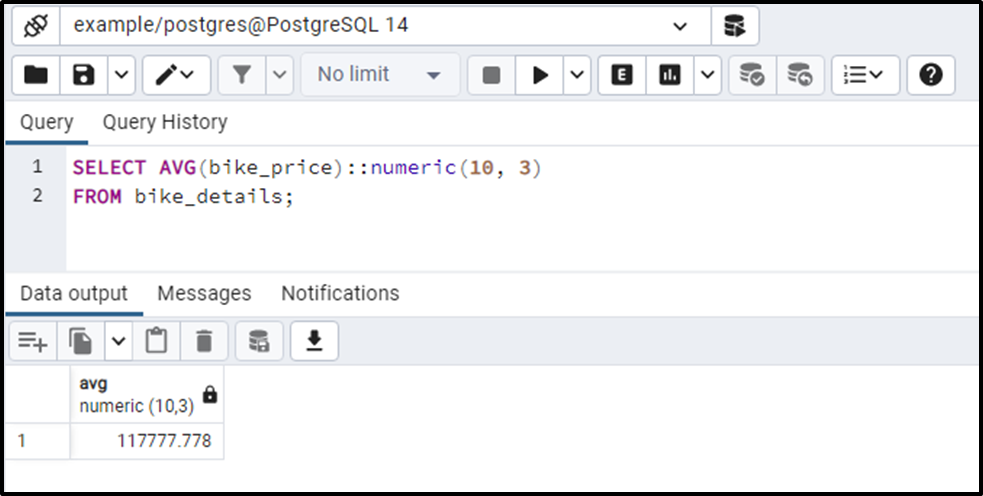Output proves the working of the AVG() function.

How to Use MAX() Function in Postgres?

PostgreSQL offers a built-in MAX() function that is used to retrieve the maximum value of a set. The below snippet will show you the basic syntax of the MAX() function:

`MAX(exp);`

Here, exp represents an expression. Let’s practically implement the MAX() function to get profound knowledge about it.

Example: How to Use MAX() Function on Table’s Data?

Let’s find the bike with the maximum price using the MAX() Function:

```SELECT MAX(bike_price)
FROM bike_details;```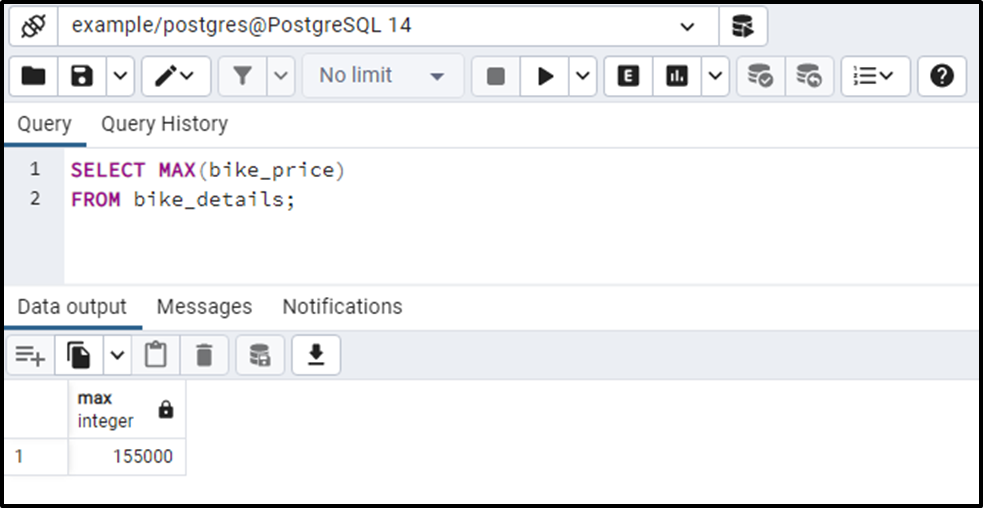The output shows that in the bike_details table, the most expensive bike costs 150000.

How Does the MIN() Function Work in Postgres?

PostgreSQL offers a built-in MIN() function that retrieves a set's minimum value. The below snippet will show you the basic syntax of the MIN() function:

`MIN(exp);`

Here, exp represents an expression. Let's implement it practically to understand the MIN() function in a better way.

Example: How to Use the MIN() Function in Postgres?

Let’s find the bike with the minimum price. To do that, we will use the Postgres MIN() Function:

```SELECT MIN(bike_price)
FROM bike_details;```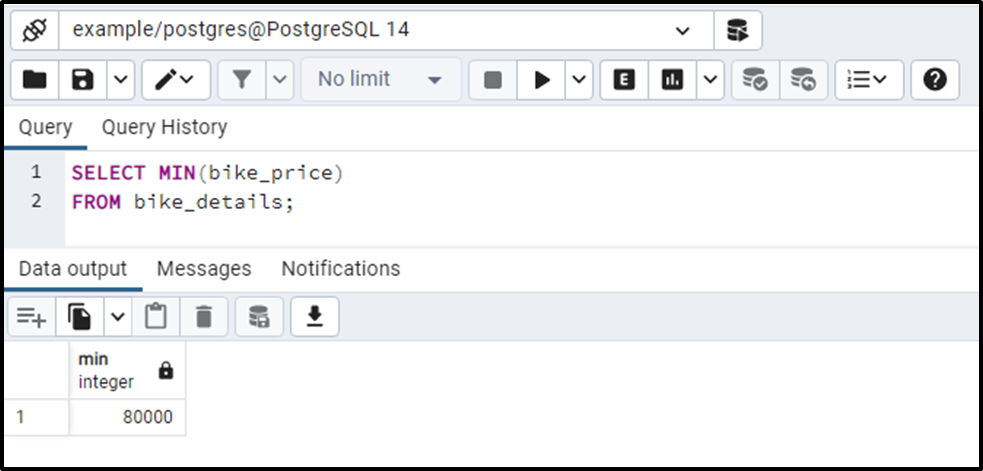The output shows that the bike_details table's most cost-effective bike costs 80000.

Conclusion

In PostgreSQL, we can perform computations/calculations on a set of rows using Postgres aggregate functions. These functions perform calculations on the table rows and return only a single row. This post explained the working of SUM(), COUNT(), AVG(), MAX(), and MIN() functions using practical examples.